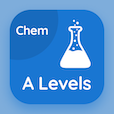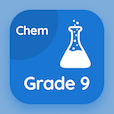A Level Courses Online

A Level Chemistry Exam Prep

A Level Chemistry Practice Test 299

The Book Chemical Formula and Equations Multiple Choice Questions and Answers (MCQs), chemical formula and equations quiz answers PDF chapter 19-299 to study online courses, a level chemistry tests. Study Moles and Equations MCQ trivia questions, chemical formula and equations Multiple Choice Questions (MCQ Quiz) for online college degrees. The eBook Chemical Formula and Equations MCQs App Download: chemical formula and equations, electron pair repulsion and bond angles, introduction to alcohols, introduction to energy changes, sulfuric acid and properties test prep for free online college classes.

The MCQ: When two aqueous solutions react together to give off solid product, this reaction is known as PDF, "Chemical Formula and Equations" App Android & iOS (Free) with oxidation, precipitation reaction, reduction, and redox reaction choices for ACT practice test. Practice moles and equations questions and answers, Google eBook to download free sample for colleges that offer online degrees.

## Chemistry: Chemical Formula and Equations MCQ Quiz

MCQ: When two aqueous solutions react together to give off solid product, this reaction is known as

A) precipitation reaction
B) oxidation
C) reduction
D) redox reaction

MCQ: The arrangement of Boron trifluoride (BF3) molecules are

A) linear
B) tetrahedral
C) hexagonal
D) trigonal

MCQ: Organic molecules with similar relative molecular mass have alcohols (CnH2n+1OH) bearing high

A) boiling point
B) polarity
C) volatility
D) Sublimation point

MCQ: The endothermic chemical reaction absorbs energy from

A) surrounding
B) inside
C) reactants
D) products

MCQ: One of the most important manufactured chemical is

A) sulfuric acid
B) nitric acid
C) benzoic acid
D) acetic acid

### Mock Tests: A Level Chemistry Course Prep

Download A level Chemistry Quiz App, SAT Chemistry MCQs App and 9th Grade Chemistry MCQ App for Android & iOS devices. These Apps include complete analytics of real time attempts with interactive assessments. Download Play Store & App Store Apps & Enjoy 100% functionality with subscriptions!